### 读取数据

import numpy as np
import os
def read_cifar10(filepath):
import pickle
def unpickle(file):
with open(file, 'rb') as fo:
file_dict = pickle.load(fo, encoding='bytes')
return file_dict
train_data = []
train_labels = []
for i in range(1, 6):
file_dict = unpickle(os.path.join(filepath, 'data_batch_%d'%i))
train_data.append(file_dict[b'data'])
train_labels += file_dict[b'labels']
train_data = np.concatenate(train_data).astype(np.float64)
file_dict = unpickle(os.path.join(filepath, 'test_batch'))
test_data, test_labels = file_dict[b'data'].astype(np.float64), file_dict[b'labels']

return train_data.reshape(train_data.shape, 3, 32, 32), np.array(train_labels), test_data.reshape(test_data.shape, 3, 32, 32), np.array(test_labels)

train_data, train_labels, test_data, test_labels = read_cifar10('../data/cifar-10-batches-py')


import matplotlib.pyplot as plt
# Visualize some examples from the dataset.
# We show a few examples of training images from each class.
classes = ['plane', 'car', 'bird', 'cat', 'deer', 'dog', 'frog', 'horse', 'ship', 'truck']
num_classes = len(classes)
samples_per_class = 7
for y, cls in enumerate(classes):
idxs = np.flatnonzero(train_labels == y)
idxs = np.random.choice(idxs, samples_per_class, replace=False)
for i, idx in enumerate(idxs):
plt_idx = i * num_classes + y + 1
plt.subplot(samples_per_class, num_classes, plt_idx)
plt.imshow(train_data[idx].transpose((1, 2, 0)).astype('uint8'))
plt.axis('off')
if i == 0:
plt.title(cls)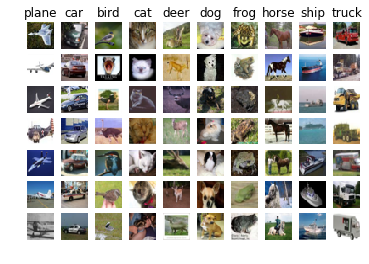### 距离度量

#### L1 (Manhattan) distance

$$d _ { 1 } \left( I _ { 1 } , I _ { 2 } \right) = \sum _ { p } \left| I _ { 1 } ^ { p } - I _ { 2 } ^ { p } \right|$$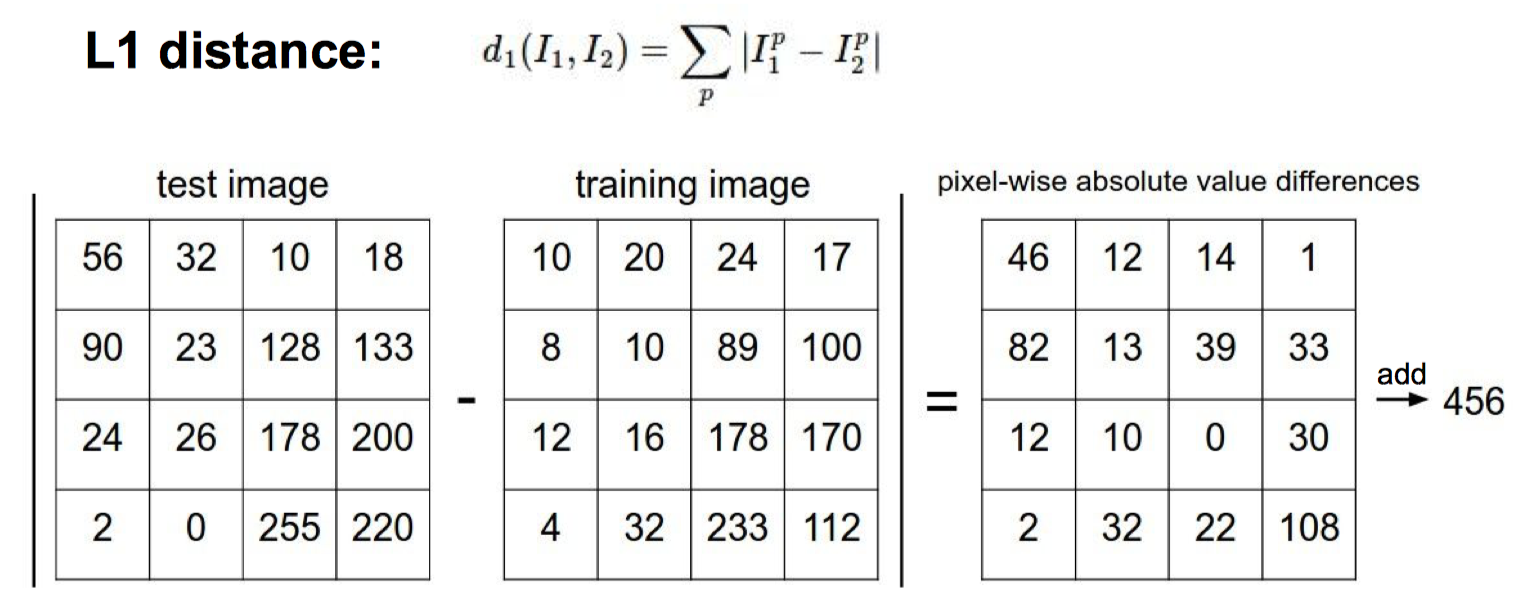L1 距离计算两个矩阵的差，取绝对值之后求所有元素的和。

test_img = test_data
train_img = train_data
np.sum(np.abs(test_img - train_img))

188615.0


#### L2 (Euclidean) distance

$$d _ { 2 } \left( I _ { 1 } , I _ { 2 } \right) = \sqrt { \sum _ { p } \left( I _ { 1 } ^ { p } - I _ { 2 } ^ { p } \right) ^ { 2 } }$$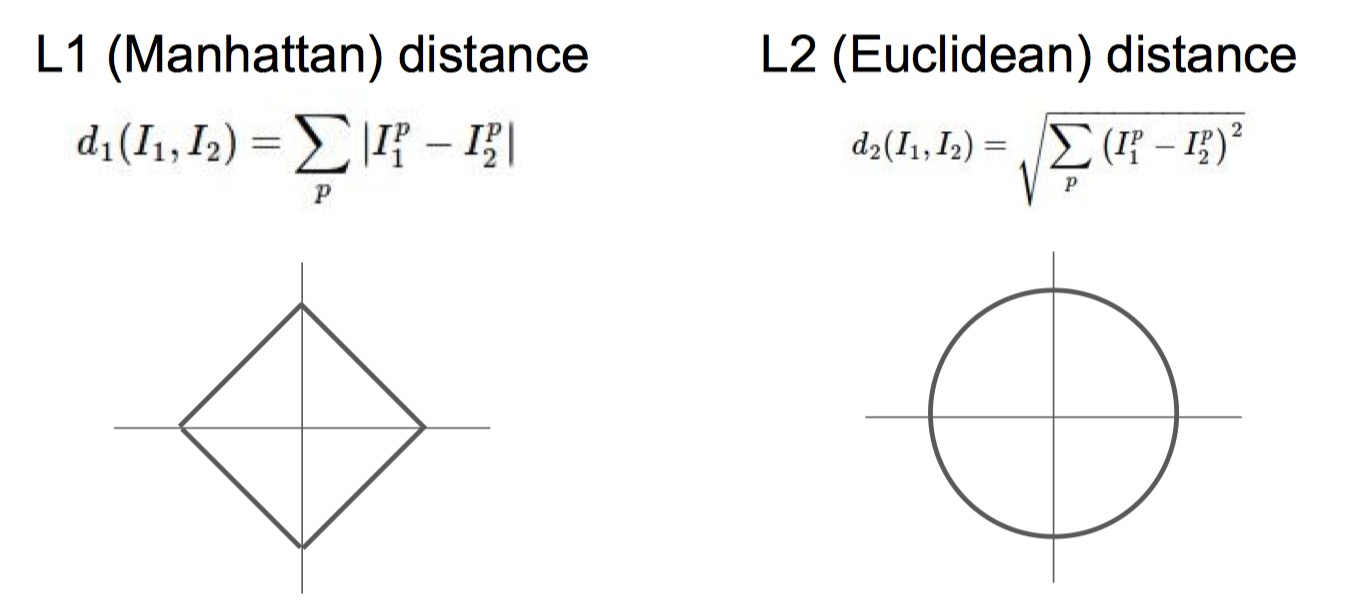### K-Nearest Neighbor Classifier

Nearest Neighbor classifier（最邻近分类器）只是简单的计算测试图片和每一张训练样本之间的距离，然后选出和测试样本距离最小的训练样本的标签作为输出。

train_labels[np.argmin(np.sum(np.abs(train_data-test_img), axis=(1, 2, 3)))]

4


The kNN classifier consists of two stages:

• During training, the classifier takes the training data and simply remembers it
• During testing, kNN classifies every test image by comparing to all training images and transfering the labels of the k most similar training examples
• The value of k is cross-validated

# Subsample the data for more efficient code execution in this exercise
num_training = 5000
train_data = train_data[:num_training].reshape((num_training, -1))
train_labels = train_labels[:num_training]
num_test = 500
test_data = test_data[:num_test].reshape((num_test, -1))
test_labels = test_labels[:num_test]
print(train_data.shape, train_labels.shape, test_data.shape, test_labels.shape)

(5000, 3072) (5000,) (500, 3072) (500,)


import numpy as np

class KNearestNeighbor(object):
""" a kNN classifier with L2 distance """

def __init__(self):
pass

def train(self, X, y):
"""
训练过程记录数据
"""
self.X_train = X
self.y_train = y

def predict(self, X, k=1, num_loops=0):
"""
预测标签
输入 (num_test, D) 数据，k 邻近，和训练数据和测试数据计算距离循环嵌套个数。
返回预测标签 list
"""
if num_loops == 0:
dists = self.compute_distances_no_loops(X)
elif num_loops == 1:
dists = self.compute_distances_one_loop(X)
elif num_loops == 2:
dists = self.compute_distances_two_loops(X)
else:
raise ValueError('Invalid value %d for num_loops' % num_loops)

return self.predict_labels(dists, k=k)

def compute_distances_two_loops(self, X):
"""
返回距离矩阵（欧氏距离）。
"""
num_test = X.shape
num_train = self.X_train.shape
dists = np.zeros((num_test, num_train))
for i in range(num_test):
for j in range(num_train):
#####################################################################
dists[i, j] = np.sum(np.square((X[i] - self.X_train[j])))
#####################################################################
return dists

def compute_distances_one_loop(self, X):
"""
Compute the distance between each test point in X and each training point
in self.X_train using a single loop over the test data.

Input / Output: Same as compute_distances_two_loops
"""
num_test = X.shape
num_train = self.X_train.shape
dists = np.zeros((num_test, num_train))
for i in range(num_test):
#######################################################################
dists[i, :] = np.sum(np.square(X[i] - self.X_train), axis=1)
#######################################################################
return dists

def compute_distances_no_loops(self, X):
"""
Compute the distance between each test point in X and each training point
in self.X_train using no explicit loops.

Input / Output: Same as compute_distances_two_loops
"""
num_test = X.shape
num_train = self.X_train.shape
#         dists = np.zeros((num_test, num_train))
#########################################################################
# 矩阵乘法 L2 = X*X^T
test_squared_sum = np.sum(np.square(X), axis=1)
test_squared_sum = np.broadcast_to(test_squared_sum.reshape(-1, 1), (num_test, num_train))
train_squared_sum = np.sum(np.square(self.X_train), axis=1)
train_squared_sum = np.broadcast_to(train_squared_sum.reshape(1, -1), (num_test, num_train))
dists = test_squared_sum + train_squared_sum - 2*np.dot(X, self.X_train.T)
#########################################################################
return dists

def predict_labels(self, dists, k=1):
"""
输入距离矩阵然后根据 K 值返回标签 list
"""
num_test = dists.shape
classes = 10
# 每一类的出现次数统计
y_count = np.zeros((num_test, classes)) # 10 类
# 原来的解法太 low 了
# 排序，获得前 k 个的下标
labels = np.argsort(dists)[:, :k].reshape(1, -1)
labels = self.y_train[labels].reshape(num_test, -1)
# 统计
for i in range(classes):
y_count[:, i] = np.sum(labels==i, axis=1)
# 获得出现次数最多的标签作为预测
y_pred = np.argmax(y_count, axis=1)
return y_pred


#### 双层循环

classifier = KNearestNeighbor()
classifier.train(train_data, train_labels)

dists = classifier.compute_distances_two_loops(test_data)
print(dists.shape)

(500, 5000)


np.sum(train_labels[np.argmin(dists, axis=1)]==test_labels)/500

0.274


%matplotlib inline
plt.rcParams['figure.figsize'] = (10.0, 8.0) # set default size of plots
plt.rcParams['image.interpolation'] = 'nearest'
plt.rcParams['image.cmap'] = 'gray'

plt.imshow(dists, interpolation='none')
plt.show()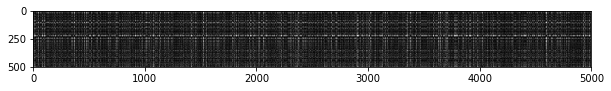Inline Question #1: Notice the structured patterns in the distance matrix, where some rows or columns are visible brighter. (Note that with the default color scheme black indicates low distances while white indicates high distances.)

• What in the data is the cause behind the distinctly bright rows?
• What causes the columns?

y_test_pred = classifier.predict_labels(dists, k=1)
# Compute and print the fraction of correctly predicted examples
num_correct = np.sum(y_test_pred == test_labels)
accuracy = float(num_correct) / test_labels.shape
print('Got %d / %d correct => accuracy: %f' % (num_correct, num_test, accuracy))

Got 137 / 500 correct => accuracy: 0.274000


y_test_pred = classifier.predict_labels(dists, k=5)
num_correct = np.sum(y_test_pred == test_labels)
accuracy = float(num_correct) / num_test
print('Got %d / %d correct => accuracy: %f' % (num_correct, num_test, accuracy))

Got 139 / 500 correct => accuracy: 0.278000


Inline Question 2 We can also other distance metrics such as L1 distance. The performance of a Nearest Neighbor classifier that uses L1 distance will not change if (Select all that apply.):

1. The data is preprocessed by subtracting the mean.
2. The data is preprocessed by subtracting the mean and dividing by the standard deviation.
3. The coordinate axes for the data are rotated.
4. None of the above.

#### 单层循环

# Now lets speed up distance matrix computation by using partial vectorization
# with one loop. Implement the function compute_distances_one_loop and run the
# code below:
dists_one = classifier.compute_distances_one_loop(test_data)

# To ensure that our vectorized implementation is correct, we make sure that it
# agrees with the naive implementation. There are many ways to decide whether
# two matrices are similar; one of the simplest is the Frobenius norm. In case
# you haven't seen it before, the Frobenius norm of two matrices is the square
# root of the squared sum of differences of all elements; in other words, reshape
# the matrices into vectors and compute the Euclidean distance between them.
difference = np.linalg.norm(dists - dists_one, ord='fro')
print('Difference was: %f' % (difference, ))
if difference < 0.001:
print('Good! The distance matrices are the same')
else:
print('Uh-oh! The distance matrices are different')

Difference was: 0.000000
Good! The distance matrices are the same


#### 无循环

L2 范数为:

$$\sum(X-Y)^2\\ =\sum X^2+\sum Y^2-2XY$$

# Now implement the fully vectorized version inside compute_distances_no_loops
# and run the code
dists_two = classifier.compute_distances_no_loops(test_data)

# check that the distance matrix agrees with the one we computed before:
difference = np.linalg.norm(dists - dists_two, ord='fro')
print('Difference was: %f' % (difference, ))
if difference < 0.001:
print('Good! The distance matrices are the same')
else:
print('Uh-oh! The distance matrices are different')

Difference was: 0.000000
Good! The distance matrices are the same


# Let's compare how fast the implementations are
def time_function(f, *args):
"""
Call a function f with args and return the time (in seconds) that it took to execute.
"""
import time
tic = time.time()
f(*args)
toc = time.time()
return toc - tic

two_loop_time = time_function(classifier.compute_distances_two_loops, test_data)
print('Two loop version took %f seconds' % two_loop_time)

one_loop_time = time_function(classifier.compute_distances_one_loop, test_data)
print('One loop version took %f seconds' % one_loop_time)

no_loop_time = time_function(classifier.compute_distances_no_loops, test_data)
print('No loop version took %f seconds' % no_loop_time)

# you should see significantly faster performance with the fully vectorized implementation

Two loop version took 39.697376 seconds
One loop version took 56.282101 seconds
No loop version took 0.357180 seconds


cs231n 的 knn 实现有一个坑，数据类型必须是 float64，否则会超出数据可表示范围，我建议先除个 255。

#### Cross-validation（交叉验证）

num_folds = 5
k_choices = [1, 3, 5, 8, 10, 12, 15, 20, 50, 100]

# X_train_folds = []
# y_train_folds = []
################################################################################
# TODO:                                                                        #
# Split up the training data into folds. After splitting, X_train_folds and    #
# y_train_folds should each be lists of length num_folds, where                #
# y_train_folds[i] is the label vector for the points in X_train_folds[i].     #
# Hint: Look up the numpy array_split function.                                #
################################################################################
# nums_per_fold = train_data.shape//num_folds
# for i in range(num_folds-1):
#     X_train_folds.append(train_data[nums_per_fold*i:nums_per_fold*(i+1), :])
#     y_train_folds.append(train_labels[nums_per_fold*i:nums_per_fold*(i+1)])
# X_train_folds.append(train_data[nums_per_fold*(i+1):, :])
# y_train_folds.append(train_labels[nums_per_fold*(i+1):])

X_train_folds = np.split(train_data, num_folds, axis=0)
y_train_folds = np.split(train_labels, num_folds)

################################################################################
#                                 END OF YOUR CODE                             #
################################################################################

# A dictionary holding the accuracies for different values of k that we find
# when running cross-validation. After running cross-validation,
# k_to_accuracies[k] should be a list of length num_folds giving the different
# accuracy values that we found when using that value of k.
k_to_accuracies = {}

################################################################################
# TODO:                                                                        #
# Perform k-fold cross validation to find the best value of k. For each        #
# possible value of k, run the k-nearest-neighbor algorithm num_folds times,   #
# where in each case you use all but one of the folds as training data and the #
# last fold as a validation set. Store the accuracies for all fold and all     #
# values of k in the k_to_accuracies dictionary.                               #
################################################################################

for k in k_choices:
for i in range(num_folds):
cur_train_data = np.concatenate(X_train_folds[:i]+X_train_folds[i+1:])
cur_train_labels = np.concatenate(y_train_folds[:i]+y_train_folds[i+1:])
cur_test_data = X_train_folds[i]
cur_test_labels = y_train_folds[i]
if k not in k_to_accuracies.keys():
k_to_accuracies[k] = []
classifier.train(cur_train_data, cur_train_labels)
y_test_pred = classifier.predict(cur_test_data, k=k, num_loops=0)
num_correct = np.sum(y_test_pred == cur_test_labels)
accuracy = float(num_correct) / test_labels.shape
k_to_accuracies[k].append(accuracy)

################################################################################
#                                 END OF YOUR CODE                             #
################################################################################

# Print out the computed accuracies
for k in sorted(k_to_accuracies):
for accuracy in k_to_accuracies[k]:
print('k = %d, accuracy = %f' % (k, accuracy))

k = 1, accuracy = 0.526000
k = 1, accuracy = 0.514000
k = 1, accuracy = 0.528000
k = 1, accuracy = 0.556000
k = 1, accuracy = 0.532000
k = 3, accuracy = 0.478000
k = 3, accuracy = 0.498000
k = 3, accuracy = 0.480000
k = 3, accuracy = 0.532000
k = 3, accuracy = 0.508000
k = 5, accuracy = 0.496000
k = 5, accuracy = 0.532000
k = 5, accuracy = 0.560000
k = 5, accuracy = 0.584000
k = 5, accuracy = 0.560000
k = 8, accuracy = 0.524000
k = 8, accuracy = 0.564000
k = 8, accuracy = 0.546000
k = 8, accuracy = 0.580000
k = 8, accuracy = 0.546000
k = 10, accuracy = 0.530000
k = 10, accuracy = 0.592000
k = 10, accuracy = 0.552000
k = 10, accuracy = 0.568000
k = 10, accuracy = 0.560000
k = 12, accuracy = 0.520000
k = 12, accuracy = 0.590000
k = 12, accuracy = 0.558000
k = 12, accuracy = 0.566000
k = 12, accuracy = 0.560000
k = 15, accuracy = 0.504000
k = 15, accuracy = 0.578000
k = 15, accuracy = 0.556000
k = 15, accuracy = 0.564000
k = 15, accuracy = 0.548000
k = 20, accuracy = 0.540000
k = 20, accuracy = 0.558000
k = 20, accuracy = 0.558000
k = 20, accuracy = 0.564000
k = 20, accuracy = 0.570000
k = 50, accuracy = 0.542000
k = 50, accuracy = 0.576000
k = 50, accuracy = 0.556000
k = 50, accuracy = 0.538000
k = 50, accuracy = 0.532000
k = 100, accuracy = 0.512000
k = 100, accuracy = 0.540000
k = 100, accuracy = 0.526000
k = 100, accuracy = 0.512000
k = 100, accuracy = 0.526000

# plot the raw observations
for k in k_choices:
accuracies = k_to_accuracies[k]
plt.scatter([k] * len(accuracies), accuracies)

# plot the trend line with error bars that correspond to standard deviation
accuracies_mean = np.array([np.mean(v) for k,v in sorted(k_to_accuracies.items())])
accuracies_std = np.array([np.std(v) for k,v in sorted(k_to_accuracies.items())])
plt.errorbar(k_choices, accuracies_mean, yerr=accuracies_std)
plt.title('Cross-validation on k')
plt.xlabel('k')
plt.ylabel('Cross-validation accuracy')
plt.show()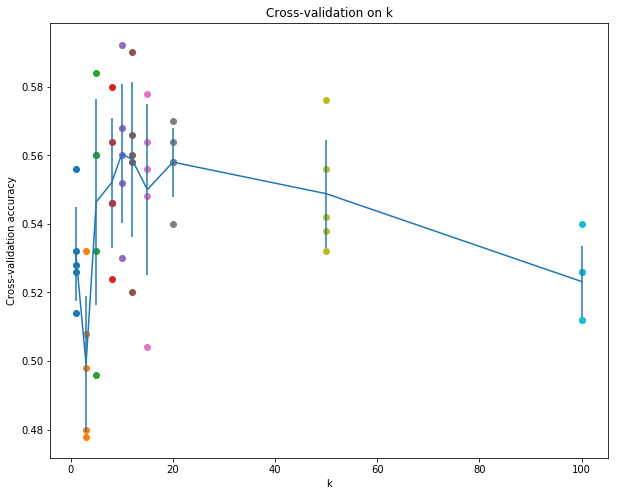# Based on the cross-validation results above, choose the best value for k,
# retrain the classifier using all the training data, and test it on the test
# data. You should be able to get above 28% accuracy on the test data.
best_k = 10

classifier = KNearestNeighbor()
classifier.train(train_data, train_labels)
y_test_pred = classifier.predict(test_data, k=best_k)

# Compute and display the accuracy
num_correct = np.sum(y_test_pred == test_labels)
accuracy = float(num_correct) / num_test
print('Got %d / %d correct => accuracy: %f' % (num_correct, num_test, accuracy))

Got 141 / 500 correct => accuracy: 0.282000


Inline Question 3 Which of the following statements about $k$-Nearest Neighbor ($k$-NN) are true in a classification setting, and for all $k$? Select all that apply.

1. The training error of a 1-NN will always be better than that of 5-NN.
2. The test error of a 1-NN will always be better than that of a 5-NN.
3. The decision boundary of the k-NN classifier is linear.
4. The time needed to classify a test example with the k-NN classifier grows with the size of the training set.
5. None of the above.

L2 距离时 k-NN 不是线性的。只有 4 是对的，k-NN 需要和每一个训练样本进行比较。